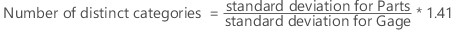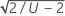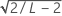Methods and formulas for number of distinct categories in Nested Gage R&R Study

Select the method or formula of your choice.

Number of distinct categories

The number of distinct categories represents the number of non-overlapping confidence intervals that will span the range of product variation. You can also think of it as the number of groups within your process data that your measurement system can discern.Minitab then truncates this value, except when the value is less than 1. In that case, Minitab sets the number of distinct categories equal to 1.

Confidence interval

Suppose L and U are the lower and upper bounds of the ratio of the gage variance and the total variance, then the lower and the upper bounds for the number of distinct categories are:Note

L and U must be in (0, 1). If L and U are outside the range, the lower and upper bounds for the number of distinct categories are missing.

By using this site you agree to the use of cookies for analytics and personalized content.  Read our policy